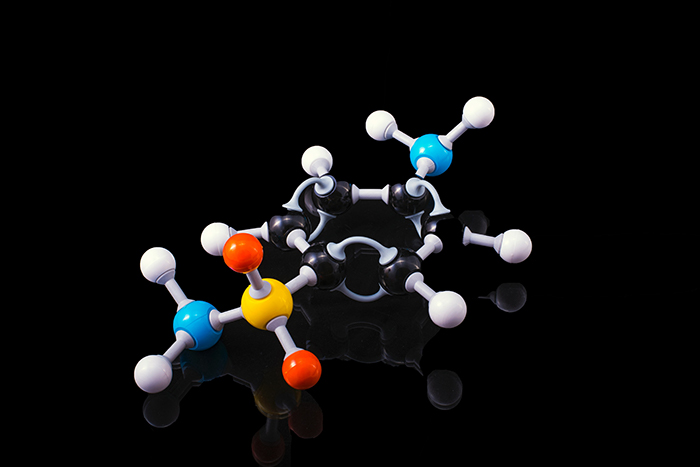# AP Chemistry Prep

Prerequistes

Basic Chemistry Sr. High

Basic Chemistry Jr. High

Pre Calculus

course information
Course Focus:
Chemistry
Start Date:
November 29, 2021
End Date:
February 14, 2022
Number of weeks:
9
Student Information
Target Skill Level :
AP
Day of the week:
Mondays
Instructor :
Vincenzo Angelo
Teaching Format:
In Person## Unit 1: Atomic Structure and Properties

• Moles and molar mass
• Atomic Number & Atomic Mass - What is this important?
• Valence Electrons & What that matters

## Unit 2: Molecular and Ionic Compound Structure and Properties

• Types of chemical bonds (Ionic & Covalent)
• Crystals & Lattices - what they form the shapes they do
• Lewis diagrams

## Unit 3: Inter-molecular Forces and Properties

• Solids, liquids, and gases
• Ideal gas law
• Kinetic molecular theory
• Deviation from ideal gas law
• Solutions and mixtures
• Solubility
• Beer-Lambert Law (concentration is linked to absorption)

## Unit 4: Chemical Reactions

• Introduction for reactions
• Representations of reactions
• Stoichiometry - stuff gets in the way
• Types of chemical reactions
• Introduction to acid-base reactions
• Oxidation-reduction (redox) reactions

## Unit 5: Kinetics

• Reaction rates
• Introduction to rate law
• Concentration changes over time
• Elementary reactions
• Collision model
• Reaction mechanism and rate law
• Catalysis

## Unit 6: Thermodynamics

• Endothermic and exothermic processes
• Energy diagrams
• Heat transfer and thermal equilibrium
• Heat capacity and calorimetry
• Energy of phase changes
• Introduction of enthalpy of reaction
• Bond enthalpies
• Enthalpy of formation
• Hess's Law

## Unit 7: Equilibrium

• Introduction to equilibrium
• Direction of reversible reactions
• Reaction quotient and equilibrium constant
• Calculating the equilibrium constant
• Magnitude of the equilibrium constant
• Introduction to solubility equilibria
• Common-ion effect
• pH and solubility

## Unit 8: Acids and Bases

• Introduction to acids and bases
• pH and pOH of strong acids and bases
• Weak acid and base equilibria
• Acid-base reactions and buffers
• Acid-base titrations
• Molecular structures of acids and bases
• pH and pKa
• Properties of buffers
• Henderson-Hasselbalch equation
• Buffer capacity

## Unit 9: Applications of Thermodynamics

• Introduction to entropy
• Absolute entropy and entropy change
• Gibbs Free Energy and thermodynamic favorability
• Thermodynamic and kinetic control
• Free energy and equilibrium